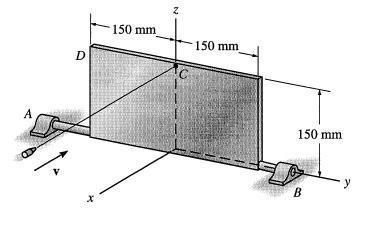## Angular momentum and velocity question, help

tbone
Posts: 1
Joined: Fri Apr 25, 2008 7:02 am

### Angular momentum and velocity question, help

Hi, this is a question i came across and have been struggling with, some help would be great!

The 15kg plate shown is able to rotate on its axle which is supported on the journal
bearings at A and B. A bullet is fired into the plate providing it with an initial
angular momentum of 9kgm/s. Find the angular velocity of the plate when it has
rotated through 180 degrees from the position shown. (Neglect the mass of the bullet
compared with that of the plate).The main problem i have is im not sure how to get the moment of inertia, im pretty sure i have to work it out about the y axis shown. im not sure if i have to take into account position vectors and such either. anyway any help on where to get started and what equations i need to use would be great.

Thanks

marten
Posts: 134
Joined: Thu Aug 30, 2007 10:21 am

### Re: Angular momentum and velocity question, help

Well, as a tip to get started, check out the parallel axis thereom.

Marten

twistor
Posts: 1529
Joined: Thu Apr 13, 2006 2:47 pm

### Re: Angular momentum and velocity question, help

That question is a little too engineering oriented for my tastes.

blueeverest
Posts: 38
Joined: Fri Mar 28, 2008 3:09 pm

### Re: Angular momentum and velocity question, help

is that the linear momentum of the bullet at impact or what? I think the units of angular momentum are multiplied by meters.

blueeverest
Posts: 38
Joined: Fri Mar 28, 2008 3:09 pm

### Re: Angular momentum and velocity question, help

Assuming that initial L=9, and applying conservation of angular momentum (180 degrees doesnt really matter), I found angular velocity=80.

moment of inertia about side b for a*b rectangular plate is ma^2/3. Yess, it doesnt depend on side b.

butsurigakusha
Posts: 293
Joined: Sun Oct 07, 2007 8:05 pm

### Re: Angular momentum and velocity question, help

180 degrees matters because there is a torque applied by gravity as it rotates.

I used conservation of energy and got 82 s^-1, which is just a slight correction to the answer that didn't take gravity into account.

1/2 * I * wf^2 = 1/2 * I * wi^2 + m*g*h

blueeverest
Posts: 38
Joined: Fri Mar 28, 2008 3:09 pm

### Re: Angular momentum and velocity question, help

okay, that makes sense. i see what u mean.
thanks.

quizivex
Posts: 1031
Joined: Tue Jan 09, 2007 6:13 am

### Re: Angular momentum and velocity question, help

wow, that question was apparently a bit tricker than it looked. very astute observation, butsu...

little things like the gravity torque in this problem are the kinds of things we often overlook in GRE problems and get questions wrong because of it... reminds me of a textbook problem that asked us to calculate the final velocity of a positively charged ball that was dropped from a height onto a large negatively charged table... we had just learned the formula for the E field of a plane, and the interesting fact that it's uniform... so all we thought about when solving the problem was the electrostatic energy... and we forgot that gravitational energy was still present.